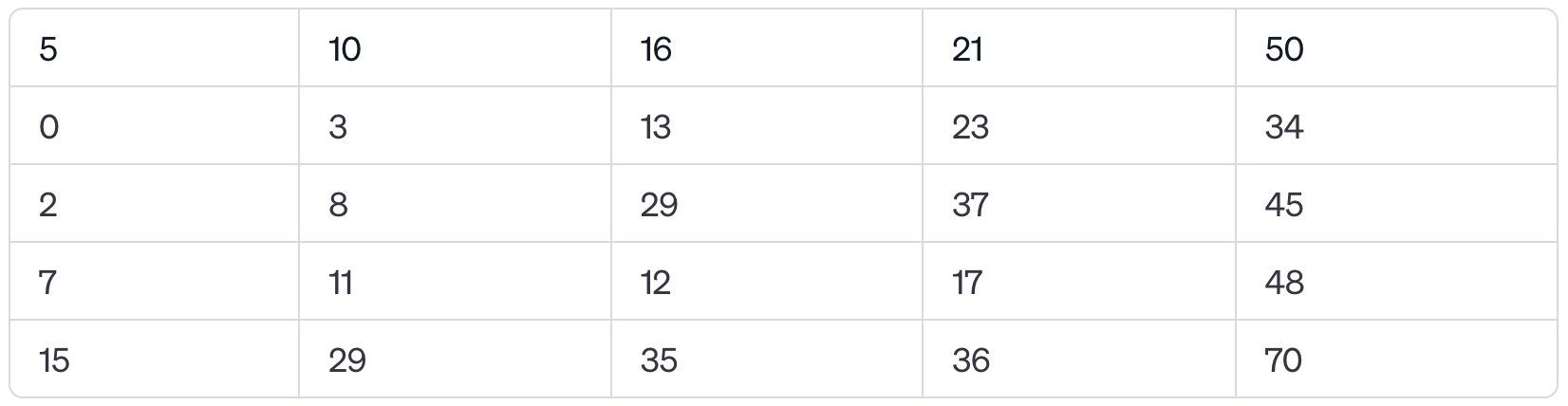Login or Create an Account to view the mark scheme, comment, and add to a test
The following table represents a 2D array called SEMISORTED where each row contains elements sorted in ascending order. The rows are not sorted in relation to each other.(a).
State the value of SEMISORTED

(b).
Construct an algorithm that outputs every element in the 2D array SEMISORTED.

(c).
Modify the algorithm in b) to efficiently search the 2D array SEMISORTED for a given number K. If K is present output TRUE, otherwise output FALSE.

(d).
Suggest how the 2D array SEMISORTED might be more efficiently searched using a combination of linear and binary search. Also indicate the characteristic of the 2D array SEMISORTED data that makes this optimisation convenient.


HomeThe Right Test - Fast